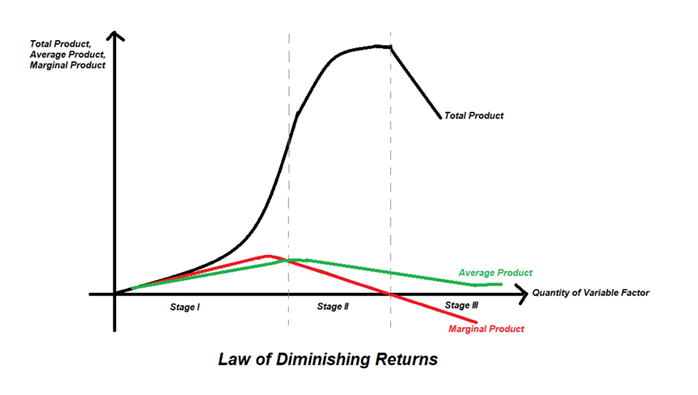# Diminishing Returns

• Updated on

## What is meant by diminishing returns?

Diminishing returns is also known as the law of diminishing returns or law of diminishing marginal productivity, or the law of variable proportions. The factors of production may be classified as fixed or variable. As per the law, holding all other factors constant, when more and more of a variable input is employed, initially the production increases at an increasing rate. Later the production increases at a decreasing rate until finally when the output begins to decline. This law can be understood based on the following concepts- total product, average product and marginal product.

Summary
• The law of diminishing returns studies how marginal product begins to decline as more and more of a variable factor are employed to a set of constant fixed factors.
• This law is studied with the assumptions of constant technology, variable factor combinations and only one variable factor.
• The stages of production are divided into three phases- first where marginal product increases, second where marginal product decreases and third where the marginal product becomes negative.

## What is meant by total product, average product and marginal product?

Total product is the total output produced with the total inputs employed. While the average product is the output per unit of the variable factor employed. The average product is estimated using the following formula-

Average Product = Total Product/ Number of units of variable factor employed

Marginal product, on the other hand, shows the additional output produced by employing an additional unit of the variable factor. The law of diminishing returns studies how marginal product begins to decline as more and more of a variable factor are employed to a set of constant fixed factors. Marginal product is estimated using the formula

Marginal product = Change in total product/ Change in amount of variable factor employed.

## What are the assumptions behind this law?

For this law to hold good, the following assumptions are required

• Holding state of technology constant.
• Only one factor is varied while all others are fixed.
• Using variable factor combination or proportions.
• All units of the variable factor are homogeneous
• The observations are for a short period.

## What are the stages in which we observe diminishing returns?

Diminishing returns to a variable factor can be studied in three stages, namely- increasing returns to a variable factor, diminishing returns to a variable factor and negative returns to a variable factor.• The first stage or the stage of increasing returns to a factor

As more and more factors are initially employed, the total product first increases at an increasing rate and then begins to grow at a diminishing rate. When the total product increases at an increasing rate, the marginal product increases, and so does the average product. Then when the total product begins to grow at a diminishing rate, the marginal product begins to fall. However, when the marginal product begins to decline in this stage, the average product is still increasing and is lesser than the value of the marginal product. It may be noted that the point where the total product changes its rate of increase is called the point of inflection. At the point of inflection, the marginal product is at its peak.

• The second stage or the stage diminishing returns to a factor.

With further employment of the variable factor, when production enters stage two, the total product grows but at a diminishing rate. Correspondingly marginal product declines but is still positive. The starting point of Stage two is where the average product is at its peak and is beginning to fall. Now the value of the average product is greater than that of the marginal product. Stage two of production ends where the total product reaches its maximum level.

• The third stage or the stage of negative returns to a factor

In the third stage, the total product stays at its peak for a while and then declines- this means that with each additional unit of labour employed, the output falls further and further. As a result, marginal product is zero when the total product remains constant, and then with a fall in the total product, marginal product value goes into negative values. However, the average product continues to decline but is positive in value.

## What causes increasing marginal returns to a factor in the initial stages?

Initially, when variable factors are employed, the fixed factor is in abundance and is not completely utilised. So as more and more variable factors work on them, there is a better utilisation of the fixed resources, and thus, there are increasing returns. Also, when the variable factor is available in abundance, there is scope for specialisation or division of labour resulting in higher productivity.

## What causes diminishing marginal returns to a factor?

Once the variable factor is sufficient to ensure efficient utilisation of the fixed factors, employment of more variable factors makes the fixed factors relatively lower, leading to a decline in average productivity and marginal productivity beginning to decline. This is because every additional variable factor can increase the total output by lesser and lesser amounts. The fixed factors are characterised by indivisibility. Thus, when they are put to use more and more intensively, the factor proportions are lower on optimality. The diminishing returns is also caused because factors are not perfect substitutes for each other.

Labour is a variable factor working on a farm land